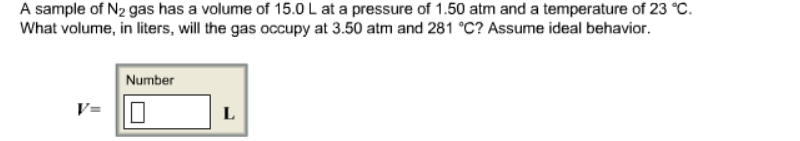# Problem: A sample of N2 gas has a volume of 15.0 L at a pressure of 1.50 atm and a temperature of 23°C. What volume, in liters, will the gas occupy at 3.50 atm and 281°C? Assume ideal behavior. V =

###### FREE Expert Solution###### Problem Details

A sample of N2 gas has a volume of 15.0 L at a pressure of 1.50 atm and a temperature of 23°C. What volume, in liters, will the gas occupy at 3.50 atm and 281°C? Assume ideal behavior.

V =